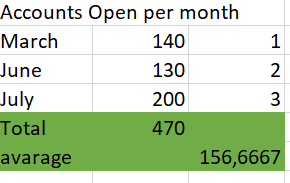## calculate average using the data part (Month)

Good day expects.

i want to calculate the average of the open accounts in a month using the below sas script

``Expected outcome``
Spoiler```proc sql;
create table want as
select sum(account) / (datepart (date_opened,'month')) as avg
/*date_opened ,AccNrSer*/
from work.have ;
quit;```
9 REPLIES 9

## Re: calculate average using the data part (Month)

What is wrong with the code you show? How is this avarage [sic] computed?

--
Paige Miller

## Re: calculate average using the data part (Month)

the code is not giving me the expected results

## Re: calculate average using the data part (Month)

Please @Rixile106 notice that I already asked a question about your expected results, which has not been answered. Saying "does not give me the expected results" does not in any way indicate what you do expect. We need to know what you do expect in order to write code.

--
Paige Miller

## Re: calculate average using the data part (Month)

DATEPART, given a SAS datetime value will return a date value, which is the number of days since 01JAN1960 and is almost certainly not involved in any reasonable way with calculating an "average". I expect that you are getting errors because datepart expects something that resolves to a numeric value and I doubt that (date_opened,'month') qualifies.

If you were wanting a month from the date then perhaps: month(datepart(date_opened)) but there is no way that dividing by a month number makes sense.

GROUPBY the calendar month perhaps. But using just Month leaves you subject to dates across years being counted as one.

```proc sql;
create table want as
select sum(account) / (datepart (date_opened,'month')) as avg
/*date_opened ,AccNrSer*/
from work.have ;
quit;```

Provide some example data if you expect a working solution and the shown expected outcome is not a data set, or is very poorly structured on. A REPORT perhaps.

## Re: calculate average using the data part (Month)

date_open is a numeric field.
below is an example of a data set

account date_open
56611781 20220131
76254189 20220131
2504589 20220231
2508862 20221231
115234141 20211231
680315210014 20221001
680914510015 202191101
680914510016 202130125
680914510014 202130125

## Re: calculate average using the data part (Month)

@Rixile106 wrote:
date_open is a numeric field.
below is an example of a data set

account date_open
56611781 20220131
76254189 20220131
2504589 20220231
2508862 20221231
115234141 20211231
680315210014 20221001
680914510015 202191101
680914510016 202130125
680914510014 202130125

Explaining the problem to us should not be as difficult as you are making it. Your original problem had a variable called "Accounts Open Per Month", where is that? How can we provide code if the data you just showed does not connect with the original problem statement? Whatever you tell us needs to be consistent with the original problem statement.

In SAS, 20221001 is not a date. It is simply a big integer 20,221,001 that has no calendar related meaning. If you want to treat this as a year/month/date, you can create a valid SAS date value like this (in a DATA step)

``````yearmonth=input(put(date_open,8.),yymmdd8.);
format yearmonth monyy7.;``````

or in PROC SQL

``input(put(date_open,8.),yymmdd8.) as yearmonth format=monyy7.``

--
Paige Miller

## Re: calculate average using the data part (Month)

@Rixile106 wrote:
date_open is a numeric field.
below is an example of a data set

account date_open
56611781 20220131
76254189 20220131
2504589 20220231
2508862 20221231
115234141 20211231
680315210014 20221001
680914510015 202191101
680914510016 202130125
680914510014 202130125

If your date_open variable is numeric what format does it have assigned? If the format is something like BEST12. or 12. then the value is a simple number and not a date. And running datepart function against it is even more useless. See this example:

```data junk;
file print;
date_open= 20220131;
y= datepart(date_open);
put y= ;
run;```

Whic shows Y is 234. Why would you divide anything by 234 to get an "average".

Run Proc Contents on your data set and show us the result.

## Re: calculate average using the data part (Month)

I don't like this approach but it will get you what you want.

``````data have;
input account \$ date_open;
cards;
56611781 20220131
76254189 20220131
2504589 20220228
2508862 20221231
115234141 20211231
680315210014 20221001
680914510015 20191101
680914510016 20230125
680914510014 20230125
;
run;

proc sql;
create table monthly_totals as
select put(input(put(date_open, 8. -l), yymmdd8.), yymmn6.)  as Open_Month, count(distinct account) as num_accounts
from have
group by calculated open_month;
quit;

proc sql;
create table summaries as
select 'Total' as Open_Month, sum(t1.num_accounts) as num_accounts
from monthly_totals t1
union
select 'Average' as Open_Month, mean(t2.num_accounts) as num_accounts
from monthly_totals t2;
quit;

data want;
length open_month \$20.;
set monthly_totals summaries;
run;

``````

## Re: calculate average using the data part (Month)

Datepart takes a single parameter, a date variable. MONTH() as a function calculates the month from a date.

That SQL is very wrong, I would suggest showing your data (not in a spoiler or as an image) and what you're trying to calculate.

Assuming your top 3 rows are correct, to calculate the sum and average, you could use SQL such as:

proc sql;
create table want as
select sum(total) as Total, mean(total) as Average
from have;
quit;